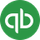cancel
Showing results for
Search instead for
Did you mean:Intuit

# Calculate gross wages

Learn how to calculate gross wages.

Grossing up a paycheck means to calculate the gross wages obtained from a specified net pay amount. We explain how to calculate gross wages.

To successfully calculate the gross from the net, you must know:

• Net amount
• Taxes to be withheld

The basic formula to gross up an amount is:
net amount + flat tax amounts / (100% - total percent of taxes) = Gross

An example

Assume the desired net pay amount is \$200, and you want to withhold social security, medicare and \$100 for federal income tax (FIT).

1. Total the tax percentages.
Social Security 6.2% + Medicare 1.45% = 7.65%
2. Subtract the total from 100%
100-7.65 = 92.35
3. Convert that number to a percentage by moving the decimal two positions to the left
0.9235
4. Add \$100 from FIT to the net
\$100 + \$200 = \$300
5. Divide the new net amount by the amount in step
300 / .9235 = 324.85
6. The gross amount to be used is \$324.85.
The net amount will be \$200 after withholding social security, Medicare, and \$100 for FIT.

Was this helpful?

You must sign in to vote, reply, or post
Sign in for the best experience
Ask questions, get answers, and join our large community of QuickBooks users.
Sign In / Sign Up

Sitemap